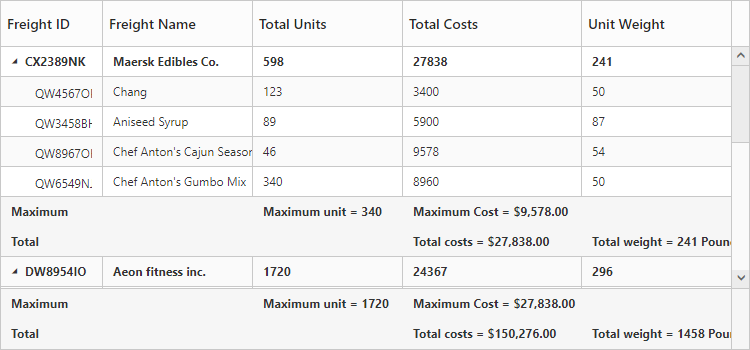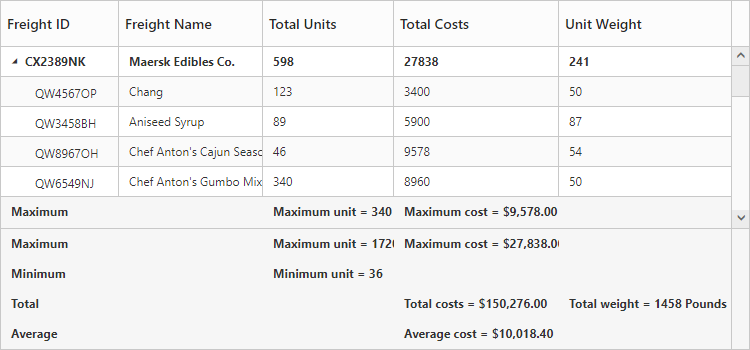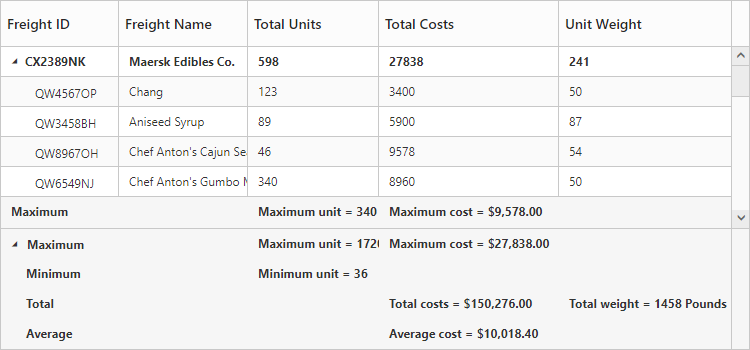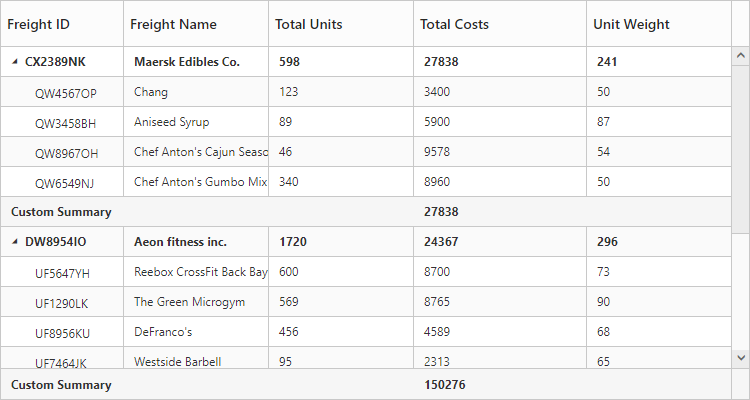# Summary Row

24 Sep 201813 minutes to read

Summary rows in TreeGrid is used to summarize every hierarchy with the set of predefined summary types using the column values.

## Defining summary columns

The below code snippet explains defining a summary row in TreeGrid,

• HTML
• ``````<ej-treegrid id="TreeGridControl" [dataSource]="treeGridData"
[showSummaryRow] = "true"
[showTotalSummary] = "true"
[summaryRows] = "summaryrows" >
//..
</ej-treegrid>``````
• JAVASCRIPT
• ``````import {Component} from '@angular/core';
@Component({
selector: 'ej-app',
templateUrl: 'app/app.component.html',
styleUrls: ['app/app.component.css']
})
export class AppComponent {
public summaryrows : any;
constructor() {
//...
this.summaryrows = [{
title: "Maximum",
summaryColumns: [
{
summaryType: ej.TreeGrid.SummaryType.Maximum,
dataMember: "TotalUnits",
displayColumn: "TotalUnits",
prefix: "Maximum unit = "
},
{
summaryType: ej.TreeGrid.SummaryType.Maximum,
dataMember: "TotalCosts",
displayColumn: "TotalCosts",
prefix: "Maximum Cost = ",
format: "{0:C}"
}
]
},
{
title: "Total",
summaryColumns: [
{
summaryType: ej.TreeGrid.SummaryType.Sum,
dataMember: "TotalCosts",
displayColumn: "TotalCosts",
prefix: "Total costs = ",
format: "{0:C}"
},
{
summaryType: ej.TreeGrid.SummaryType.Sum,
dataMember: "UnitWeight",
displayColumn: "UnitWeight",
prefix: "Total weight = ",
suffix: " Pounds"
}]
}],
}
}``````

The below screenshot shows the output of above code example.## Customize height of total summary

Using `totalSummaryHeight` property we can customize the height of the total summary container.
The below code example shows how to update the footer summary container height.

• HTML
• ``````<ej-treegrid id="TreeGridControl" [dataSource]="treeGridData"
[showTotalSummary] = "true"
[totalSummaryHeight] = totalSummaryHeight >
//..
</ej-treegrid>``````
• JAVASCRIPT
• ``````import {Component} from '@angular/core';
@Component({
selector: 'ej-app',
templateUrl: 'app/app.component.html',
styleUrls: ['app/app.component.css']
})
export class AppComponent {
constructor() {
//...
}
public totalSummaryHeight = 120;
}``````

The below screenshot shows the output of above code example.## Expand/collapse total summary row

We can expand/collapse the total summary rows in TreeGrid using following methods.

• Using Expander Icon
• Using Method

### Using Expander Icon

We can enable expander icon in total summary row by using `collapsibleTotalSummary` property. By default expander icon will be rendered in first row of 0th column in total summary rows.
Please find the below code example to enable collapsible total summary row in TreeGrid.

• HTML
• ``````<ej-treegrid id="TreeGridControl" [dataSource]="treeGridData"
[showTotalSummary] = "true"
[collapsibleTotalSummary] = "true" >
//..
</ej-treegrid>``````NOTE

We can also customize the expander icon column in total summary row by using `_summaryColumnIndex` property and `load` event.

### Using Method

Total summary rows in TreeGrid can be expanded/collapsed by using `expandCollapseTotalSummary` method.
Please find the code example to collapse the total summary rows below.

• HTML
• ``````<button (click)="expandCollapse(\$event, item)">expandCollapse</button>
<ej-treegrid id="TreeGridControl" [dataSource]="treeGridData"
[showTotalSummary] = "true"
[collapsibleTotalSummary] = "true" >
//..
</ej-treegrid>``````
• JAVASCRIPT
• ``````import {Component} from '@angular/core';
@Component({
selector: 'ej-app',
templateUrl: 'app/app.component.html',
styleUrls: ['app/app.component.css']
})
export class AppComponent {
constructor() {
//...
}
public expandCollapse(event, item) {
var treeObj = \$("#TreeGridControl").data("ejTreeGrid");
treeObj.expandCollapseTotalSummary(false);
}
}``````

## Custom Summary

Custom summary can be used to create summary values based on your required custom logic and calculations. To enable the custom summary, the summaryType should be set to ‘custom’ and the customSummaryValue property should be defined as function. After the custom calculation, the returned value will be displayed in the corresponding summary cell.

• HTML
• ``````<ej-treegrid id="TreeGridControl" showSummaryRow="true" [summaryRows]="summaryRow">
</ej-treegrid>``````
• JAVASCRIPT
• ``````import {Component} from '@angular/core';
@Component({
selector: 'ej-app',
templateUrl: 'app/app.component.html',
styleUrls: ['app/app.component.css']
})
export class DefaultComponent {
summaryRow:any;
constructor(public ganttDataService: GanttDataService) {
this.treeGridData = ganttDataService.getGanttData();
this.summaryRow=[
{
title: "Custom Summary",
summaryColumns: [{
summaryType: ej.TreeGrid.SummaryType.Custom,
customSummaryValue: function currency(args,data){
return ej.sum(data, "TotalCosts");
},
displayColumn: "TotalCosts"
}]
}
],
}
function currency(args, data) {
//ej.sum is aggregate to add data of total costs from datasource
return ej.sum(data, "TotalCosts");
}``````

The output of the tree grid with custom summary value is as follows.